## Graphs

 Question 1
Let G be a simple undirected graph. Let TD be a depth first search tree of G. Let TB be a breadth first search tree of G. Consider the following statements.
(I) No edge of G is a cross edge with respect to TD.(A cross edge in G is between two nodes neither of which is an ancestor of the other in TD.)
(II) For every edge (u,v) of G, if u is at depth i and v is at depth j in TB, then ∣i−j∣ = 1.
Which of the statements above must necessarily be true?
 A I only B II only C Both I and II D Neither I nor II
Data-Structures       Graphs       GATE 2018       Video-Explanation
Question 1 Explanation:
I. Undirected graph do not have cross edges in DFS. But can have cross edges in directed graph. Hence True.
II. Just draw a triangle ABC. Source is A. Vertex B and C are at same level at distance 1.
There is an edge between B and C too. So here |i - j| = |1 - 1| = 0. Hence, False.
 Question 2
Let G be a weighted connected undirected graph with distinct positive edge weights. If every edge weight is increased by the same value, then which of the following statements is/are TRUE?
P: Minimum spanning tree of G does not change
Q: Shortest path between any pair of vertices does not change
 A P only B Q only C Neither P nor Q D Both P and Q
Data-Structures       Graphs       GATE 2016 [Set-1]       Video-Explanation
Question 2 Explanation:
Given undirected weighted graph with distinct positive edges.
Every edge weight is increased by the same value say,P: Minimum Spanning Tree will not change ABC in both the cases.
Q: Shortest path will change because in 1st figure the path from A to C calculated as ABC but in fig.2, it is AC (Direct path).
 Question 3

Consider the weighted undirected graph with 4 vertices, where the weight of edge {i,j} is given by the entry Wij  in the matrix W.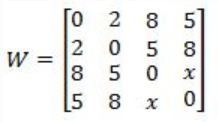The largest possible integer value of x, for which at least one shortest path between some pair of vertices will contain the edge with weight x is _________.

 A 12 B 13 C 14 D 15
Data-Structures       Graphs       GATE 2016 [Set-1]       Video-Explanation
Question 3 Explanation:
Let vertices be A, B, C and D.
x directly connects C to D.
The shortest path (excluding x) from C to D is of weight 12 (C-B-A-D).
 Question 4

In an adjacency list representation of an undirected simple graph G = (V,E), each edge (u,v) has two adjacency list entries: [v] in the adjacency list of u, and [u] in the adjacency list of v. These are called twins of each other. A twin pointer is a pointer from an adjacency list entry to its twin. If |E| = m and |V| = n, and the memory size is not a constraint, what is the time complexity of the most efﬁcient algorithm to set the twin pointer in each entry in each adjacency list?

 A Θ(n2) B Θ(n+m) C Θ(m2) D Θ(n4)
Data-Structures       Graphs       GATE 2016 [Set-2]       Video-Explanation
Question 4 Explanation:
Applying BFS on Undirected graph give you twin pointer.
Visit every vertex level-wise for every vertex fill adjacent vertex in the adjacency list. BFS take O(m+n) time.
Note:
Twin Pointers can be setup by keeping track of parent node in BFS or DFS of graph.
 Question 5

Let G be a graph with n vertices and m edges. What is the tightest upper bound on the running time of Depth First Search on G, when G is represented as an adjacency matrix?

 A θ(n) B θ(n+m) C θ(n2) D θ(m2)
Data-Structures       Graphs       GATE 2014 [Set-1]
Question 5 Explanation:
DFS visits each vertex once and as it visits each vertex, we need to find all of its neighbours to figure out where to search next. Finding all its neighbours in an adjacency matrix requires O(V ) time, so overall the running time will be O(V2).
 Question 6

Consider the directed graph given below.Which one of the following is TRUE?

 A The graph does not have any topological ordering. B Both PQRS and SRQP are topological. C Both PSRQ and SPRQ are topological orderings. D PSRQ is the only topological ordering.
Data-Structures       Graphs       GATE 2014 [Set-1]
Question 6 Explanation: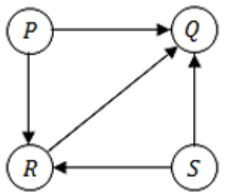There are no cycles in the graph, so topological orderings do exist.
We can consider P & S as starting vertex, followed by R & Q.
Hence, PSRQ & SPRQ are the topological orderings.
 Question 7

Consider the tree arcs of a BFS traversal from a source node W in an unweighted, connected, undirected graph. The tree T formed by the tree arcs is a data structure for computing

 A the shortest path between every pair of vertices. B the shortest path from W to every vertex in the graph. C the shortest paths from W to only those nodes that are leaves of T. D the longest path in the graph.
Data-Structures       Graphs       GATE 2014 [Set-2]
Question 7 Explanation:
One of the application of BFS algorithm is to find the shortest path between nodes u and v.
But in the given question the BFS algorithm starts from the source vertex w and we can find the shortest path from W to every vertex of the graph.
 Question 8

Suppose depth first search is executed on the graph below starting at some unknown vertex. Assume that a recursive call to visit a vertex is made only after first checking that the vertex has not been visited earlier. Then the maximum possible recursion depth (including the initial call) is _________.A 19 B 20 C 21 D 22
Algorithms       Graphs       GATE 2014 [Set-3]
Question 8 Explanation:Note: We should not consider backtrack edges, it reduces recursion depth in stack.
So the maximum possible recursive depth will be 19.Question 9

Consider a complete undirected graph with vertex set {0, 1, 2, 3, 4}. Entry Wij in the matrix W below is the weight of the edge {i, j}.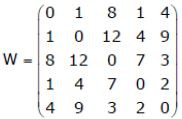What is the minimum possible weight of a path P from vertex 1 to vertex 2 in this graph such that P contains at most 3 edges?

 A 7 B 8 C 9 D 10
Data-Structures       Graphs       GATE 2010
Question 9 Explanation:The minimum possible weight of a path p from vertex 1 to vertex 2 such that p contains atmost 3 edges,= 1 + 4 + 3
= 8
 Question 10

The most efficient algorithm for finding the number of connected components in an undirected graph on n vertices and m edges has time complexity

 A θ(n) B θ(m) C θ(m+n) D θ(mn)
Data-Structures       Graphs       GATE 2008
Question 10 Explanation:
To find the number of connected components using either BFS or DFS time complexity is θ(m+n).
Suppose if we are using Adjacency matrix means it takes θ(n2).
 Question 11

The Breadth First Search algorithm has been implemented using the queue data structure. One possible order of visiting the nodes of the following graph is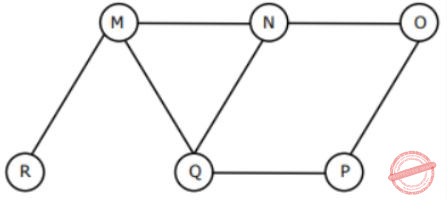A MNOPQR B NQMPOR C QMNPRO D QMNPOR
Data-Structures       Graphs       GATE 2008
Question 11 Explanation: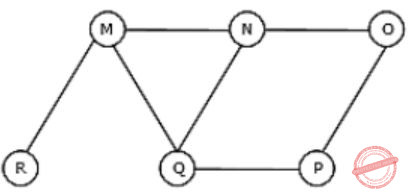Option C: QMNPRO
→ Queue starts with Q then neighbours of Q is MNP and it is matching with the given string .
→ Now , Next node to be considered is M . Neighbours of M are N, Q and R , but N and Q are already in Queue. So, R is matching with one given string
→ Now, next node to be considered is N. Neighbours of N are M, Q and O, but M and Q are already in Queue. So, O is matching with a given string.
Hence , Option C is Correct.
Similarly, check for option (D).
 Question 12

G is a graph on n vertices and 2n–2 edges. The edges of G can be partitioned into two edge-disjoint spanning trees. Which of the following is NOT true for G?

 A For every subset of k vertices, the induced subgraph has at most 2k–2 edges B The minimum cut in G has at least two edges C There are two edge-disjoint paths between every pair to vertices D There are two vertex-disjoint paths between every pair of vertices
Data-Structures       Graphs       GATE 2008
Question 12 Explanation:
→ In Spanning tree n nodes require n-1 edges. The above question they mentioned 2 disjoint spanning trees. So, it requires n-1 + n-1 = 2n-2 edges. Except option D everything is correct.
> Option A: True: Subgraph with k vertices here is no chance to get more than 2k−2 edges. Subgraph with n−k vertices, definitely less than 2n−2k edges.
-> Option B: True: Take any subgraph SG with k vertices. The remaining subgraph will have n−k vertices. Between these two subgraphs there should be at least 2 edges because we are taken 2 spanning trees in SG.
-> Option C: True: A spanning tree covers all the vertices. So, 2 edge-disjoint spanning trees in G means, between every pair of vertices in G we have two edge-disjoint paths (length of paths may vary).
 Question 13

Consider the DAG with Consider V = {1, 2, 3, 4, 5, 6}, shown below. Which of the following is NOT a topological ordering?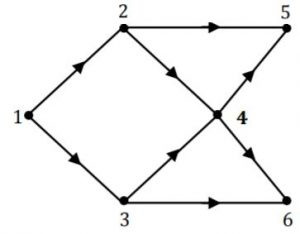A 1 2 3 4 5 6 B 1 3 2 4 5 6 C 1 3 2 4 6 5 D 3 2 4 1 6 5
Data-Structures       Graphs       GATE 2007
Question 13 Explanation:
The process to find topological order is,
(i) Go with vertex with indegree 0.
(ii) Then remove that vertex and also remove the edges going from it.
(iii) Repeat again from (i) till every vertex is completed.
Now we can see that in option (D), '3' is given first which is not possible because indegree of vertex '3' is not zero.
Hence option (D) is not topological ordering.
There are 13 questions to complete.

Register Now